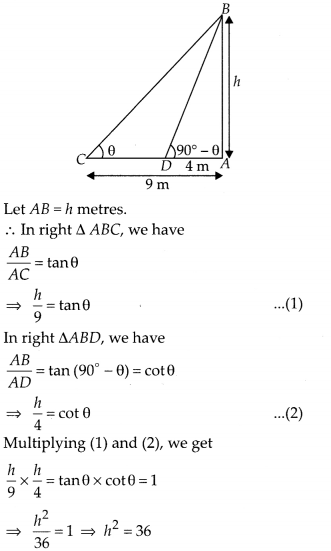# MP Board Class 10th Maths Solutions Chapter 9 Some Applications of Trigonometry Ex 9.1

In this article, we will share MP Board Class 10th Maths Book Solutions Chapter 9 Some Applications of Trigonometry Ex 9.1 Pdf, These solutions are solved subject experts from the latest edition books.

## MP Board Class 10th Maths Solutions Chapter 9 Some Applications of Trigonometry Ex 9.1

Question 1.
A circus artist is climbing a 20 m long rope, which is tightly stretched and tied from the top of a vertical pole to the ground. Find the height of the pole, if the angle by the rope with the ground level is 30° (see figure).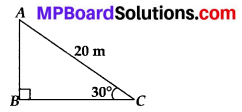Solution:
In right ∆ABC,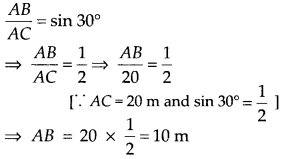Thus, the required height of the pole is 10 m.

Question 2.
A tree breaks due to storm and the broken part bends so that the top of the tree touches the ground making an angle 30° with it. The distance between the foot of the tree to the point where the top touches the ground is 8 m. Find the height of the tree.
Solution:
Let the tree is broken at A and its top is touching the ground at B.
Now, in right ∆AOB, we have
$$\frac{A O}{O B}$$ = tan 30°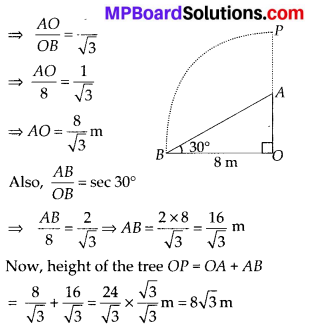Question 3.
A contractor plans to install two slides for the children to play in a park. For the children below the age of 5 years, she prefers to have a slide whose top is at a height of 1.5 m, and is inclined at an angle of 30° to the ground, whereas for elder children, she wants to have a steep slide at a height of 3 m, and inclined at angle of 60° to the ground. What should be the length of the slide in each case?
Solution:
In the figure, DE is the slide for younger children, whereas AC is the slide for elder children.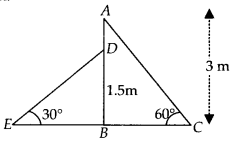In right ∆ABC, AB = 3m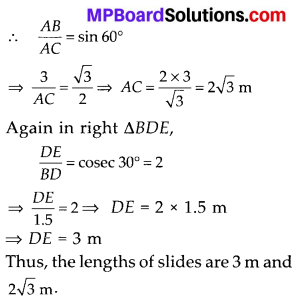Question 4.
The angle of elevation of the top of a tower from a point on the ground, which is 30 m away from the foot of the tower, is 30°. Find the height of the tower.
Solution:
In right ∆ABC, AB = height of the tower and point C is 30 m away from the foot of the tower,Question 5.
A kite is flying at a height of 60 m above the ground. The string attached to the kite is temporarily tied to a point on the ground. The inclination of the string with the ground is 60°. Find the length of the string, assuming that there is no slack in the string.
Solution:
Let OB = Length of the string
AB = 60 m = Height of the kite.
In the right ∆AOB,
∴ $$\frac{O B}{A B}$$ = cosec 60° = $$\frac{2}{\sqrt{3}}$$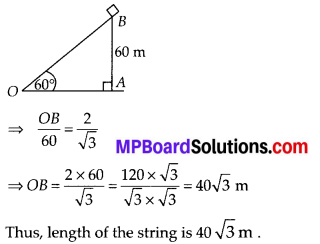Question 6.
A 1.5 m tall boy is standing at some distance from a 30 m tall building.The angle of elevation from his eyes to the top of the building increases from 30° to 60° as he walks towards the building. Find the distance he walked towards the building.
Solution:
Here, OA is the building.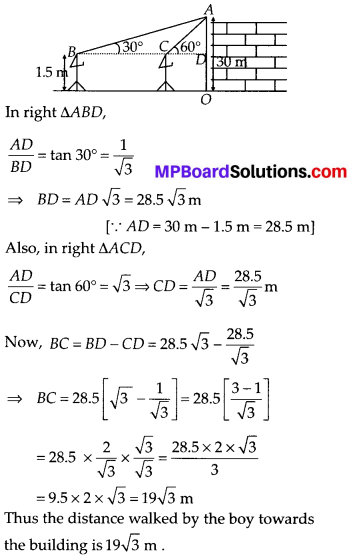Question 7.
From a point on the ground, the angles of elevation of the bottom and the top of a transmission tower fixed at the top of a 20 m high building are 45° and 60° respectively. Find the height of the tower.
Solution:
Let the height of the building be BC
∴ BC = 20 m and height of the tower be CD.
Let the point A be at a distance y metres from the foot of the building.
Now, in right ∆ABC,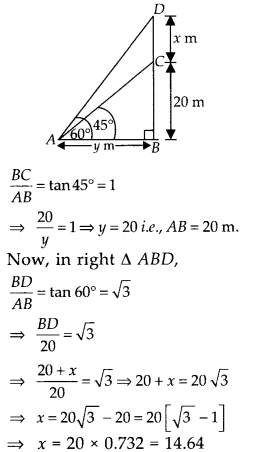Thus, the height of the tower is 14.64 m.

Question 8.
A statue, 1.6 m tall, stands on the top of a pedestal. From a point on the ground, the angle of elevation of the top of the statue is 60° and from the same point the angle of elevation of the top of the pedestal is 45°. Find the height of the pedestal.
Solution:
In the figure, DC represents the statue and BC represents the pedestal.
Now, in right ∆ABC, we have
$$\frac{A B}{B C}$$ = cot 45° = 1
⇒ $$\frac{A B}{h}$$ = 1 ⇒ AB = h metres.
Now in right ∆ABD,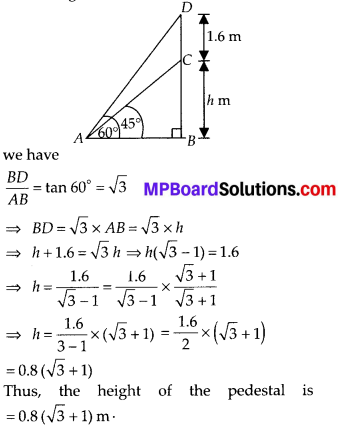Question 9.
The angle of elevation of the top of a building from the foot of the tower is 30° and the angle of elevation of the top of the tower from the foot of the building is 60°. If the tower is 50 m high, find the height of the building.
Solution:
In the figure, let height of the building = AB = h m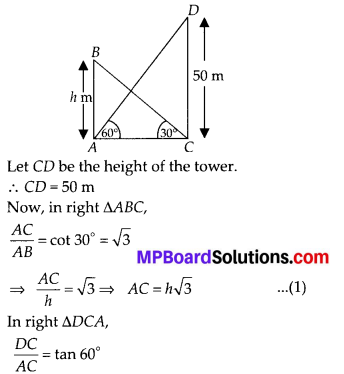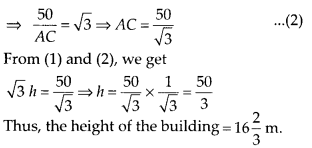Question 10.
Two poles of equal heights are standing opposite each other on either side of the road, which is 80 m wide. From a point between them on the road, the angles of elevation of the top of the poles are 60° and 30° respectively. Find the height of the poles and the distances of the point from the poles.
Solution:
Let AB = h metres = CD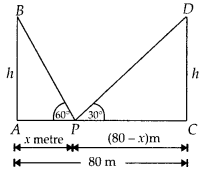and AP = x m
∴ CP = (80 – x) m
Now, in right ∆APB,
we have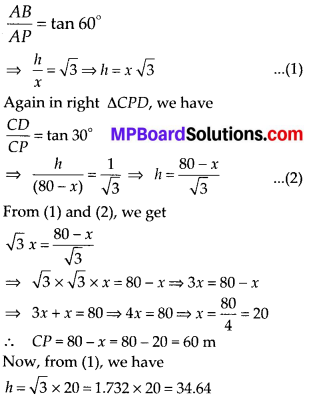Thus, the required point is 20 m away from the first pole and 60 m away from the second pole.
Height of each pole = 34.64 m.

Question 11.
A TV tower stands vertically on a bank of a canal. From a point on the other bank directly opposite the tower, the angle of elevation of the top of the tower is 60°. From another point 20 m away from this point on the line joining this point to the foot of the tower, the angle of elevation of the top of tower is 30° (see figure). Find the height of the tower and the width of the canal.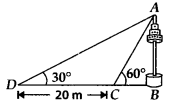Solution:
Let the TV tower be AB = h m.
Let the point ‘C be such that BC = x m and CD = 20 m.
Now, in right ∆ABC, we have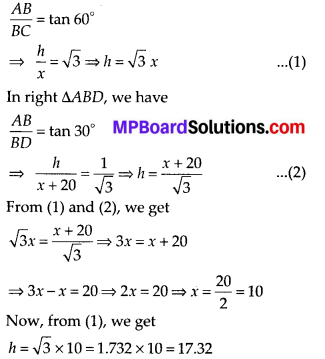Thus, the height of the tower = 17.32 m.
Also width of the canal = 10 m.Question 12.
From the top of a 7 m high building, the angle of elevation of the top of a cable tower is 60° and the angle of depression of its foot is 45°. Determine the height of the tower.
Solution:
In the figure, let AB be the height of the building.
∴ AB = 7 metres.
Let BC = X m = AE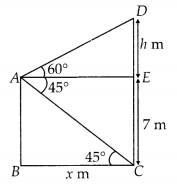Let CD be the height of the cable tower and DE = h m
∴ In right ∆DAE, we have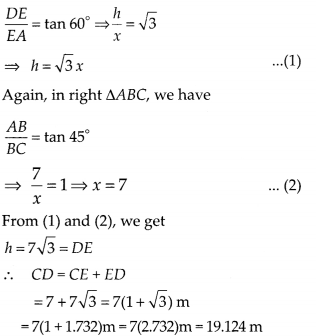Question 13.
As observed from the top of a 75 m high light house from the sea-level, the angles of depression of two ships are 30° and 45°. If one ship is exactly behind the other on the same side of the light house, find the distance between the two ships.
Solution:
In the figure, let AB represent the light house.
∴ AB = 75 m.
Let the positions of two ships be C and D such that angle of depression from A are 45° and 30° respectively.
Now, in right ∆ABC,
we have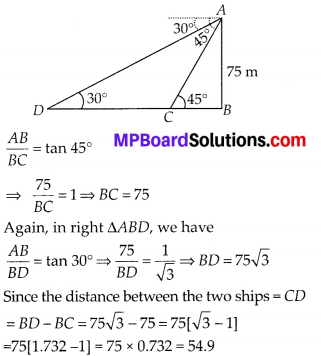Thus, the required distance between the ships is 54.9 m.

Question 14.
A 1.2 m tall girl spots a balloon moving with the wind in a horizontal line at a height of 88.2 m from the ground. The angle of elevation of the balloon from the eyes of the girl at any instant is 60°. After some time, the angle of elevation reduces to 30° (see figure). Find the distance travelled by the balloon during the interval.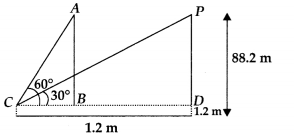Solution:
In the figure, let C be the position of the observer (the girl).
A and P are two positions of the balloon.
CD is the horizontal line from the eyes of the observer (girl).
Here PD = AB = 88.2 m – 1.2 m = 87 m
In right ∆ABC, we have
$$\frac{A B}{B C}$$ = tan 60°
⇒ $$\frac{87}{B C}=\sqrt{3}$$ ⇒ BC = $$\frac{87}{\sqrt{3}}$$ m
In right ∆PDC, we have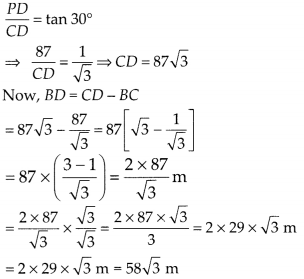Thus, the required distance between the two positions of the balloon = $$58 \sqrt{3}$$ m.Question 15.
A straight highway leads to the foot of a tower. A man standing at the top of the tower observes a car at an angle of depression of 30°, which is approaching the foot of the tower with a uniform speed. Six seconds later, the angle of depression of the car is found to be 60°. Find the time taken by the car to reach the foot of the tower from this point.
Solution:
In the figure, let AB be the height of the tower and C, D be the two positions of the car.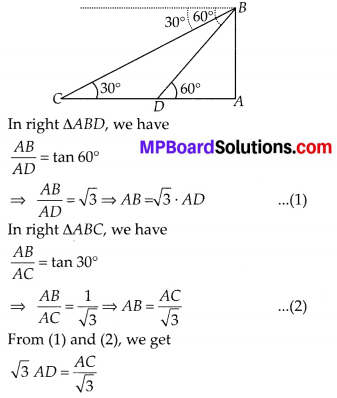⇒ AC = $$\sqrt{3} \times \sqrt{3}$$ × AD = 3 AD
∴ The distance AD will be covered in $$\frac{6}{2}$$ i.e., 3 seconds,